Excel SUMPRODUCT Function - Compute Expert

# Excel SUMPRODUCT Function

Home >> Excel Tutorials from Compute Expert >> Excel Formulas List >> Excel SUMPRODUCT Function

In this tutorial, you will learn how to use SUMPRODUCT function in excel. Excel SUMPRODUCT function has the use to multiply each digit data member in an array with its pair digit(s) in other arrays before adding up all of the multiplication results.

SUMPRODUCT function in excel is more often utilized not for its use in multiplying and adding numbers directly but for the logic evaluation of TRUE or FALSE in array before it becomes the criteria evaluation to sum numbers.

## Why We Need to Learn About SUMPRODUCT Function in Excel?

When working on data related to criteria and numbers in spreadsheet software, sometimes we might feel some difficulty and spend a lot of time when there is a need to look at how much data that we have or if we need to add numerals based on specific criteria in a table. If we use a manual process or calculating one by one on each data entry to get its total, then it will be very troublesome if there is a very vast amount of data and thus the one by one calculation process is needed to be done to get the desired result.

For case example, if we have data for hundreds or thousand company product names, maybe there is a three front letters code in the product name data entries which can be used to know the product group and we want to count how many products which are members in a particular group in the company. Or we probably want to add all the multiplication results between quantity and price to get total sales of the company. In another case, probably we want to know sales quantity from those hundreds or thousands of product names by evaluating some criteria in the product data table in the table including the previous product group. We want it because we want to get the sum of this part only to do a partial analysis, not the whole total sum.

If we use normal method to do that (probably with the help of SUM, COUNT, SUMIF/SUMIFS, and COUNT/COUNTIFS formulas too), then besides needing to calculate one by one which will spend more time, there is also a possibility that the spreadsheet will become slower in its performance if the data is very high in quantity. This is, of course, make it harder if we want to do the analysis and get the result fast.

To help with this, we can use excel SUMPRODUCT function to help the process. SUMPRODUCT function in excel takes array input in the form of cell range in its processing and can directly multiply and add up the members of the array to get the total result as desired. Excel SUMPRODUCT function is a perfect formula to be learned if we often do calculations for many numeral data with the possibility that there are a lot of criteria that needed to be considered in its processing.

## What is Excel SUMPRODUCT Function?

Excel SUMPRODUCT Function is something with the usage objective of multiplying each number in an array with its pair in other arrays before adding up all of the multiplication results. SUMPRODUCT function in excel is used much using the method of taking TRUE or FALSE evaluation of a criteria to arrays/cell ranges to count the amount of data or calculate digits in the cell range according to the criteria requirement in the input of SUMPRODUCT function in excel.

A brief explanation about the inputs of this formula is as follows:

=SUMPRODUCT(array1, array2, array3, …)

Note:
array1, array2, array3, … = all the cell ranges to be multiplied all of the contents before the result is being summed

## How to Use SUMPRODUCT Function in Excel?

The following will explain how to write an excel SUMPRODUCT function in the spreadsheet. SUMPRODUCT function in excel needs the inputs of all cell ranges that will become arrays to be multiplied each of the number pairs before the multiplication results are being summed.

Excel SUMPRODUCT function itself is usually used for three kinds of utilization: multiply and add up digits in all arrays, as usual, multiply and/or add up digits or texts that fulfill certain criteria to count the data amount, and multiply and/or add up digits or texts based on certain criteria to multiply and/or add up digit for every data entry that meets the criteria. The below part will explain how to use SUMPRODUCT function in excel based on those three kinds of excel SUMPRODUCT function utilization.

## Using Excel SUMPRODUCT Function

### Excel SUMPRODUCT Function First Utilization (Normal Multiplication and Sum)

1. Type equal sign ( = ) in the cell where you want to put the result in2. Type SUMPRODUCT (can be with large and small letters) and open bracket sign after =3. Drag cursor from the most top left to the most bottom right on the first array’s cell range and type comma sign ( , )4. Drag cursor from the most top left to the most bottom right on the second array’s cell range and type comma sign ( , ). You can also insert another comma sign and add cell range to become third array, fourth, and so on in another place until all of them are in the formula. Please note that the multiplication will be done between numbers with the same position in all of the arrays and all array inputs must be in the same size (same number of rows and columns)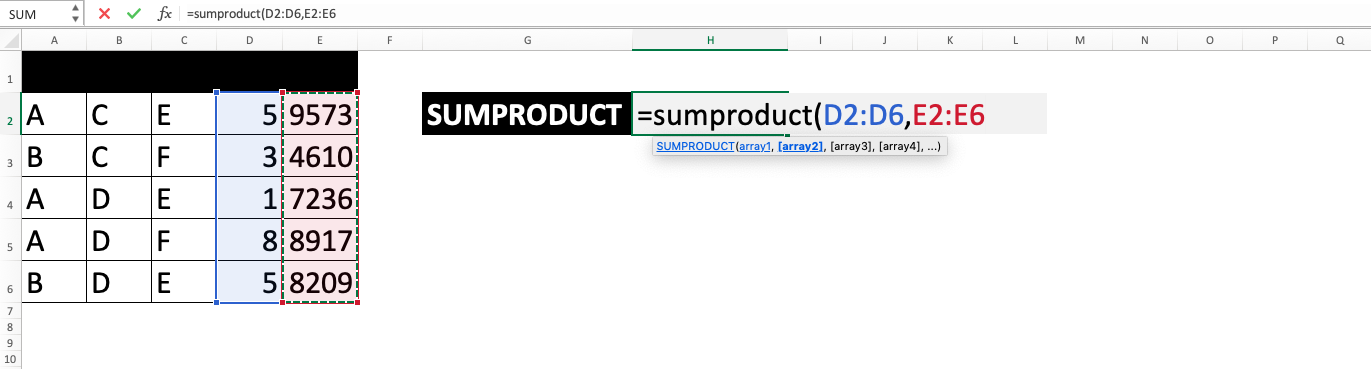5. Type close bracket sign after all cell range that you want to be processed has already been inputted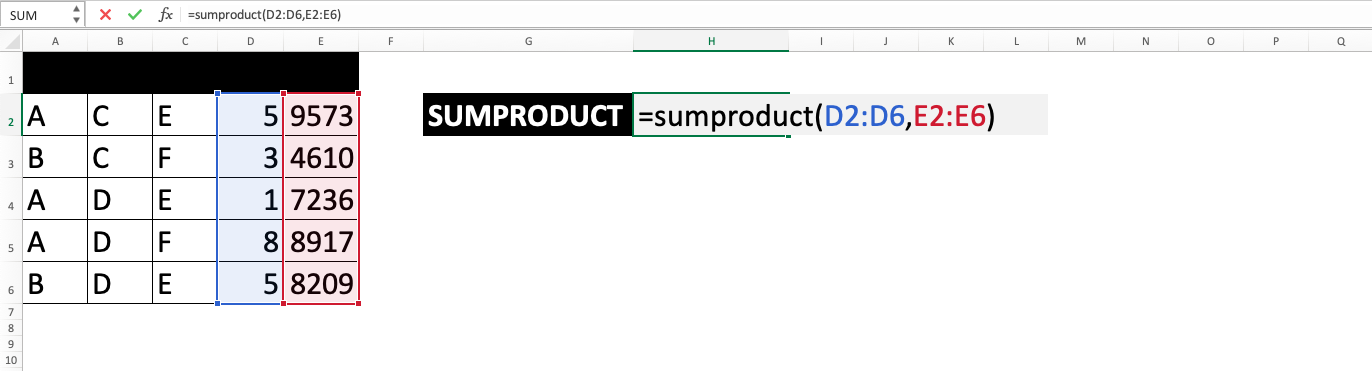6. Press Enter
7. The process of SUMPRODUCT function in excel is done!### Excel SUMPRODUCT Function Second Utilization (Multiplication and/or Sum to Count Data Amount Based on Certain Criteria(s))

1. Type equal sign ( = ) in the cell where you want to put the result in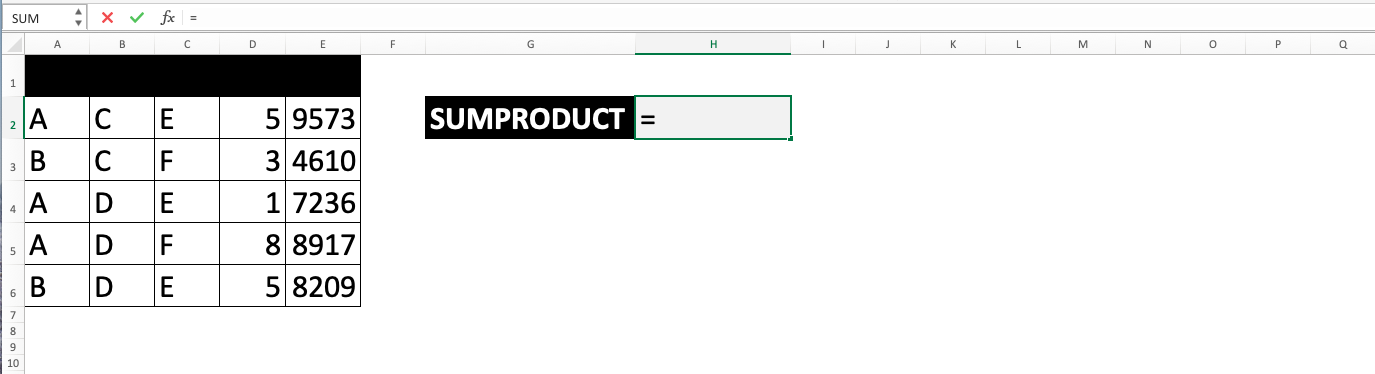2. Type SUMPRODUCT (can be with large and small letters) and open bracket sign after =3. Type minus sign ( - ) twice then type open bracket sign. These two minus signs will convert cell range to logic evaluation results of TRUE and FALSE with TRUE is represented with 1 and FALSE is represented with 04. Drag cursor from the most top left to the most bottom right on the cell range that you want to become the array5. Type the criteria for the data that you want to count the amount of in the array then type close bracket sign6. If there are still other arrays with other criteria that needs to be inputted, type comma sign ( , ) then repeat steps 3 to 5. Please note that the multiplication will be done between numbers with the same position in all of the arrays and all array inputs must be in the same size (same number of rows and columns)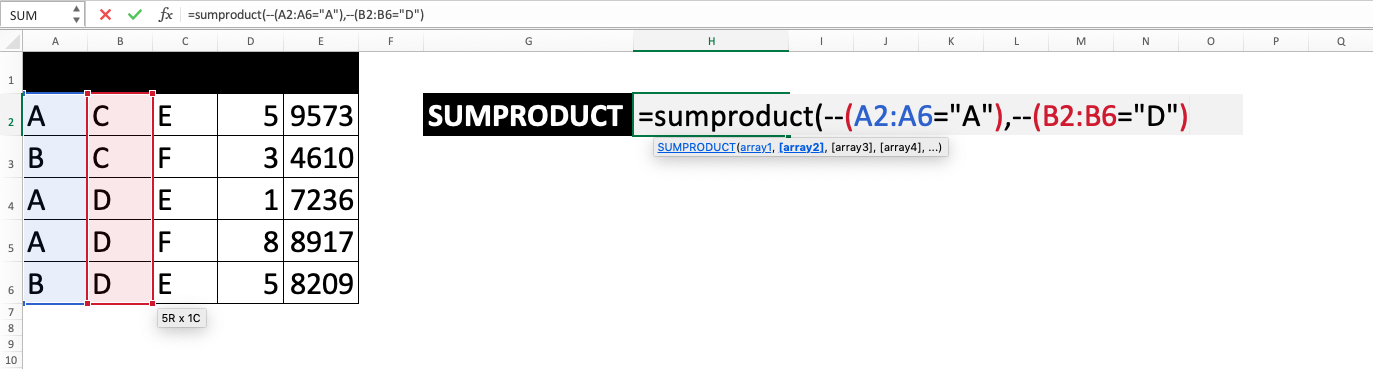7. Type close bracket sign after all cell range that you want to be processed has already been inputted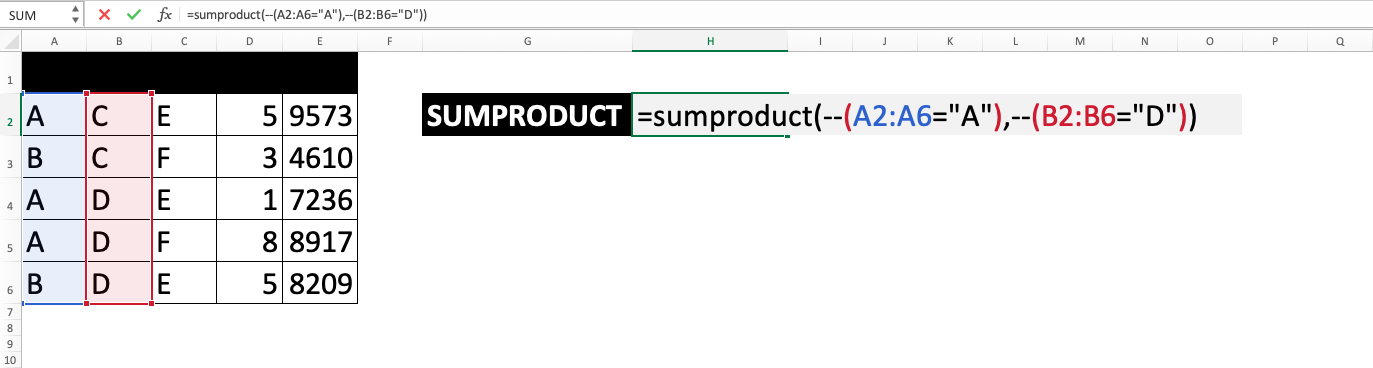8. Press Enter
9. The process of SUMPRODUCT function in excel is done!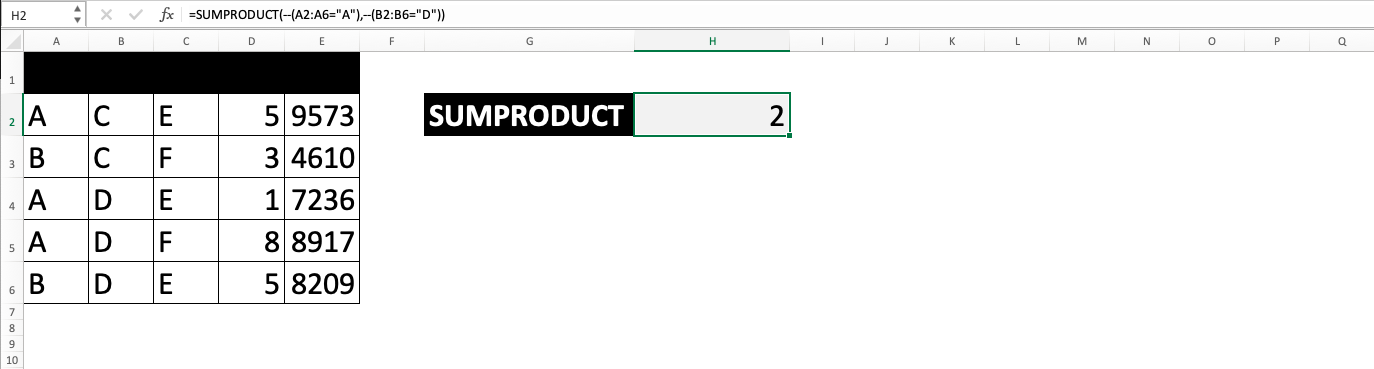Some explanations about the excel SUMPRODUCT function process: Data change on the cell range using two minus signs make the contents of it become 1 for criteria fit data and 0 for data not fit with the criteria. Because SUMPRODUCT function in excel will multiply numbers in the array with the same position of number in other arrays, then data entry that is not fit with the criteria in one of the arrays will become zero and not calculated in the count of data amount when the final sum is done by excel SUMPRODUCT function.

### Excel SUMPRODUCT Function Third Utilization (Multiplication and/or Sum to Multiply and/or Add Numbers Based on Certain Criteria(s))

1. Type equal sign ( = ) in the cell where you want to put the result in2. Type SUMPRODUCT (can be with large and small letters) and open bracket sign after =3. We will input the criteria cell range. Type minus sign ( - ) twice then type open bracket sign. These two minus signs will convert cell range to logic evaluation results of TRUE and FALSE with TRUE is represented with 1 and FALSE is represented with 04. Drag cursor from the most top left to the most bottom right on the cell range that you want to become the array5. Type the criteria for the data that you want to process the number of in the array then type close bracket sign6. If there are still other arrays with other criteria that needs to be inputted, type comma sign ( , ) then repeat steps 3 to 5. Repeat this step 6 until all of the criteria arrays have been inputted7. Type comma sign. Next, we will input the cell range where the number for the sum process will be in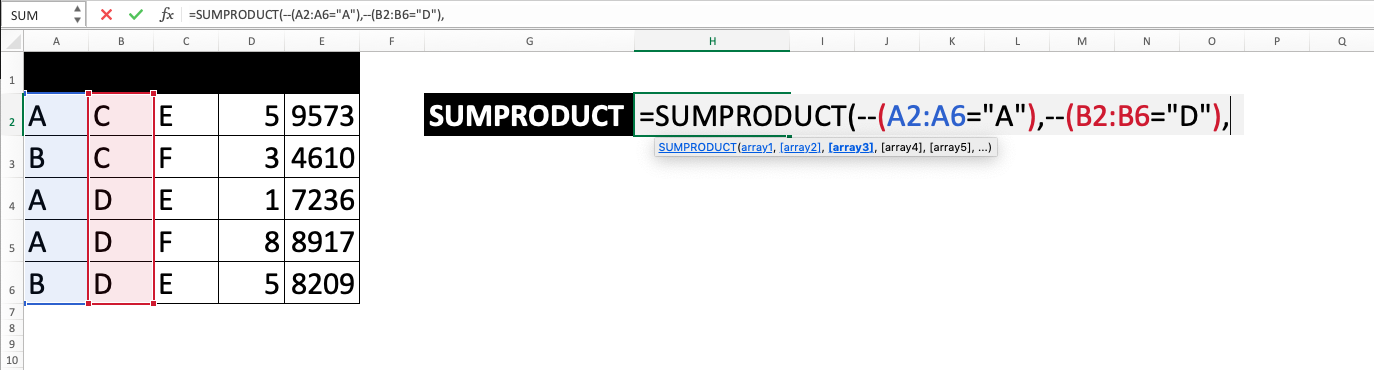8. Drag cursor from the most top left to the most bottom right on the cell range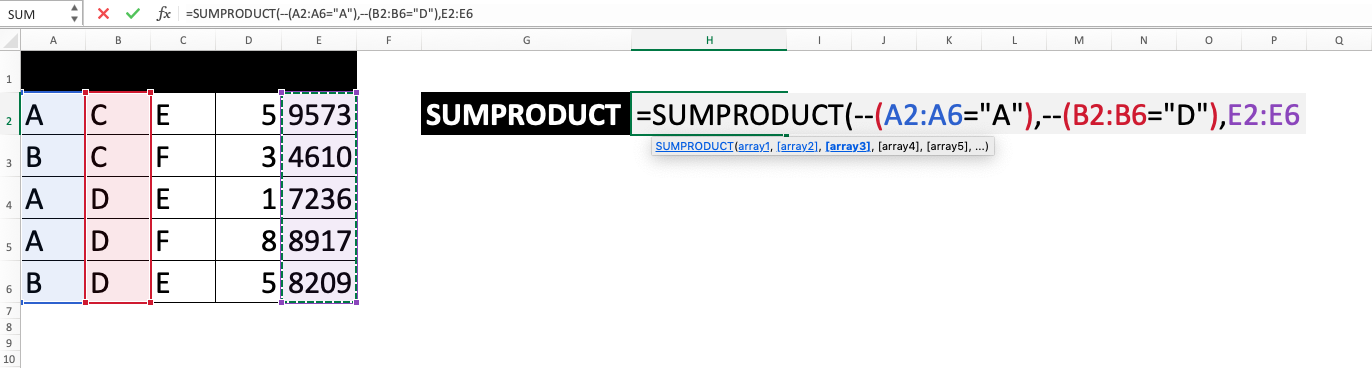9. Repeat step 7 to 8 if there is still number arrays that you want to input
10. Type close bracket sign11. Press Enter
12. The process of SUMPRODUCT function in excel is done!Some explanations about the excel SUMPRODUCT function process: Data change on the cell range using two minus signs make the contents of it become 1 for criteria fit data and 0 for data not fit with the criteria. Because SUMPRODUCT function in excel will multiply numbers in the array with the same position of number in other arrays, then data entry that is not fit with the criteria in one of the arrays will become zero and not calculated when the calculation process is done by excel SUMPRODUCT function.

## Exercise

After you learned how to do the methods’ steps from the SUMPRODUCT function in excel tutorial above, you can practice your understanding of using SUMPRODUCT function in excel through this exercise!

### Questions

Utilize excel SUMPRODUCT function to answer all questions below!
1. What is the score test 1 average of all the students?
2. How many classes got the division of A and B from the whole classes?
3. What is the score text 2 average of the students in class 2 and 3 with the C division?

• In SUMPRODUCT function in excel, non-digit data will be calculated as 0, TRUE value will be 1, and FALSE will be 0
• If you only input one cell range as an array in excel SUMPRODUCT function, then it will only sum all of the data in that array
• To increase or decrease decimal number from the result of excel SUMPRODUCT function, you can use increase decimal/decrease decimal menu from Home tab after putting the cursor in the cell where the result is.Or right click after putting the cursor to the cell where the result which decimal is wanted to be changed is, click Format Cells, and on the Number tab pick Number to then change the value on the Decimal Places based on the decimal number you want before clicking OK.But you must remember that this method only changes the decimal look of the result. If you really want to change the value, then you need to practice round function in this spreadsheet tool. The tutorial of this process is also in this blog.

Get updated excel info from Compute Expert by registering your email. It's free!